# Multilinear Kinematic Plasticity created from a stress-strain graph

I was sent a stress-strain graph of a brittle metal tested in an Instron machine. It looked like this.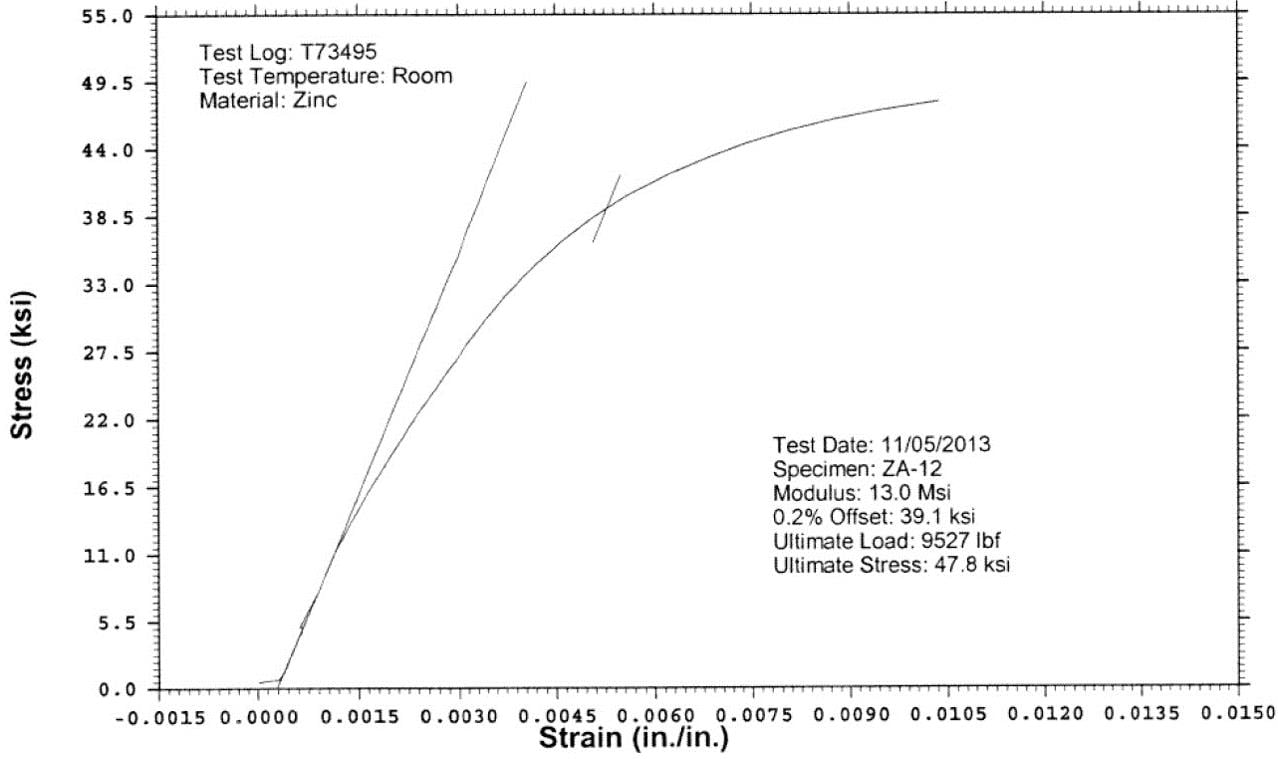I have matlab and downloaded Grabit, a utility that makes digitizing data from a graph very easy. Here is what that looked like: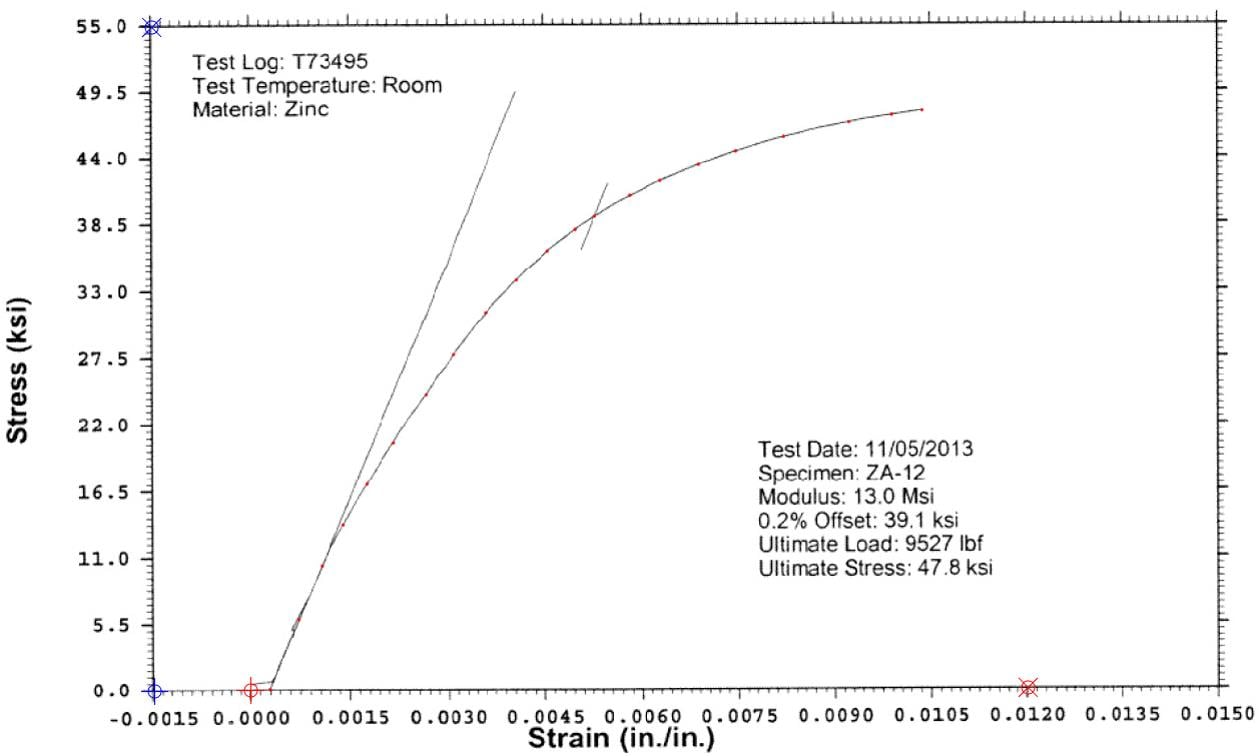Now that I had the curve digitized, I could convert the Stress and Strain into True Stress and Plastic Strain so I could enter values in a material model using Multilinear Kinematic Plasticity. The formulas to convert are shown below:

E = 13,000 ksi

True Strain = ln(1+Strain)   where ln is the natural logarithm function.

True Stress = Stress(1+Strain)

Elastic Strain = True Stress/E

Plastic Strain = True Strain - Elastic Strain

I used a spreadsheet to correct the offset in the origin of the graph to put it back on zero.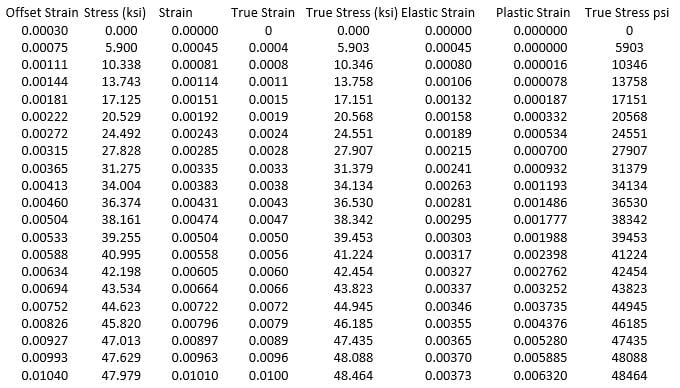The first two columns came from the matlab Grabit utility.

The last two columns are copy paste into the Multlinear Plasticity material model, except for the first line.

The first first line is the yield stress. This was the material model used for the thread fracture analysis.

«1

• edited July 2018

Peter, do you have any information like this post for strucutre steel materials?

- ASTM A572 Steel, Grade 50 (beams and columns)

- ASTM 36 (plates)

- ASTM A325 (bolts)

I found in matweb.com. But plasticity properties I did not find.

Thank you!!

• I can borrow the Atlas of Stress-Strain Curves from my Engineering Library.

Curve 6 is ASTM 36.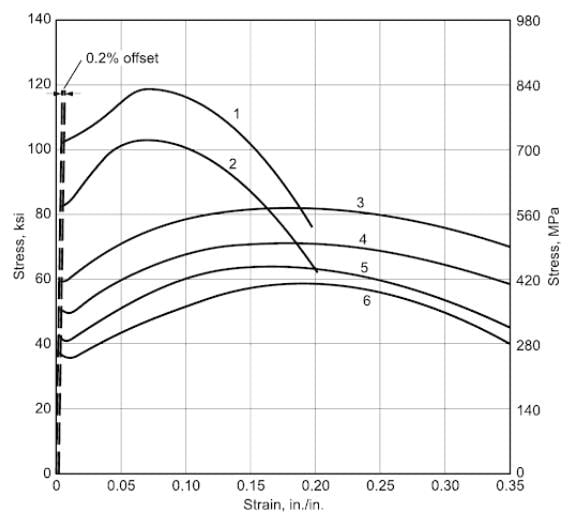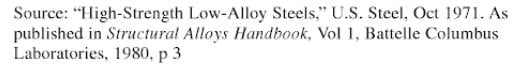• edited July 2018

Thank you, very very much Peter.

For the bolts do you have any curve?

• Fabricio,

When I search astm A325 stress strain curve on Google, it gives me this.

Figure 7 is the stress strain curve for ASTM A325.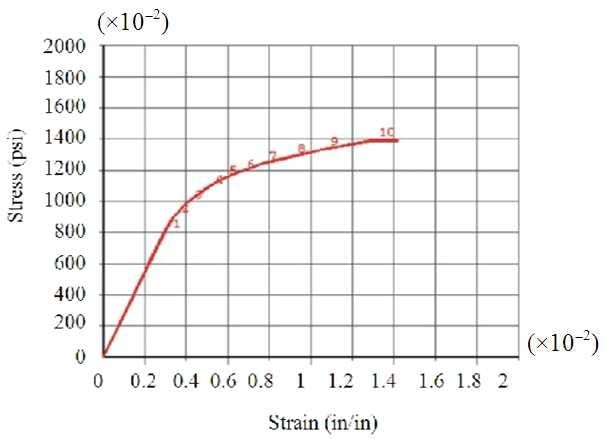You should try Google for yourself, it is really very good!

• edited July 2018

I have found a curve for bolts in this article whcih I have attached. If would like to add in your engineering library, I shared with you.

• edited July 2018

Peter I am using multilinear isotropic hardening plasticiy, but I could not see the nonlinearity.

The model is a beam, fixed in both extreme, but I modeled only the half, and use a plane of symmetry,

I could not see the nonlinear curve in equivalent total strain neither nonlinearity in the equivalent stress: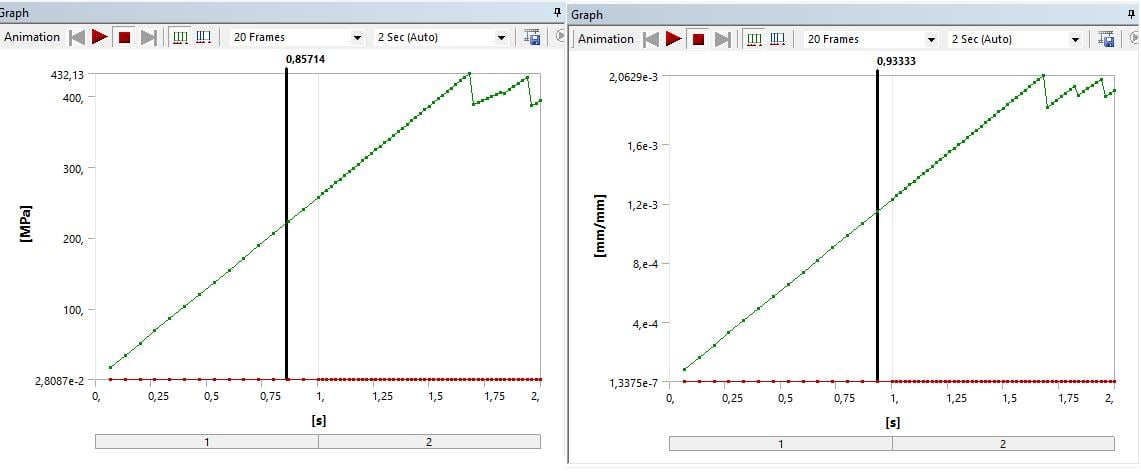In spite of reach the yeld and rupture stress, the curve is linear, I am not understanding why.

• edited July 2018
• edited July 2018

Peter, I solved. The problem was the load, which was normal to surface. So with component, the plasticity occure easier.

• edited August 2018

Peter

How you get the first and second column?

• First and second columns were digitized from the plot of Engineering Stress vs Engineering Strain.

• edited August 2018

I used Engauge Digitizier, open source, Thanks!

• For digitizing data from research articles, WebPlotDigitizer is a very good app that I used extensively in my research.

It works as an App on your Chrome browser.

Regards,

Sandeep

• edited August 2018

Hi,

the third column (Strain) , how you get?

• Third column in a Stress vs Strain plot, Can you explain or post a picture?

You can digitize any no. of curves from a 2-D plot using WebPlot Digitizer.

~Sandeep

• edited August 2018

I did not understand, too. Which column??First and second column?Third column? I did not understand nothing.

Can you explain?

Fabricio

• This post has a table of numbers in an Excel spreadsheet, the first few lines are below.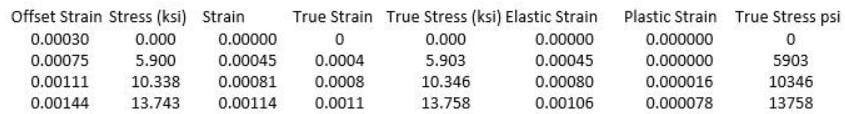Column 1 is Offset Strain and is the value of strain read off the plot (using digitizing sofware) in that same post.

Column 2 is the Stress read off the plot

Column 3 is Strain = Offset Strain - 0.0003    (which is the first value in the table).

So Columns 2 and 3 are Engineering Stress and Engineering Strain (which start at 0,0) that get converted to True Stress and True Strain and the components of True Strain, which are Elastic Strain and Plastic Strain using the formulas listed in that post.

Published sources of Stress-Stain data usually start at (0,0), but raw data straight from the Instron can have offsets that need to be corrected. I hope that clarifies things for everyone.

Regards,

Peter

• edited August 2018

Peter, it clarifies.

But the column that I have to input in Ansys is the last (True Stress) and one before (plastic strain), right?

Another point, your first column is offstet strain. In the picture below in the X axis, I have the strain, and in your material graph you have the strain, too. Why did you use offset strain? I did not understand.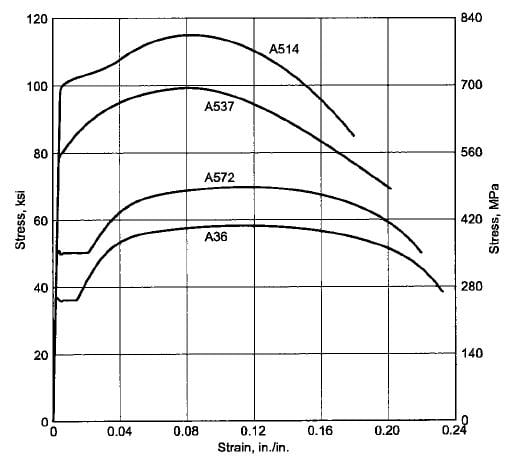I am with difficult, to input the ASTM A325 curve. Because I did not find the engineering stress. Only the true stress, and I think that is not reliable.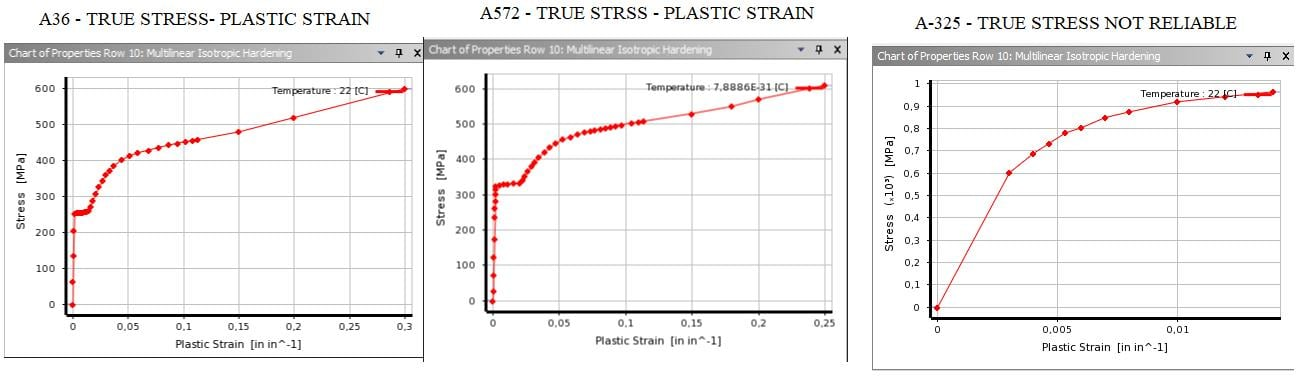I think that is not reliable, because I was reading about ASTM A325 curve, and it has a yield landing as the other two types of steel.

Can you help me with it?

• I created a column called offset strain because the plot was clearly not going through zero strain, which was at the red cursor location. The plot went through the point 0.0003 strain instead of zero due to some slack in the Instron testing setup. Therefore, the whole curve needed to shift to the left by 0.0003 to pass through zero. The column where the offset was subtracted off was called Strain, which was the Engineering Strain.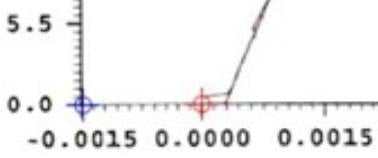• edited August 2018

Now I understood.

But I have problems with the ASTM A325 curve. Because this, the connection does not have the stiffness that it should have.

The model is attached.

• Fabricio,

I opened the spreadsheet from above and looked at the A325 tab. Here are the columns:You have left two columns off: Elastic Strain and Plastic Strain, and you don't have a cell for E, the Young's Modulus.

Elastic Strain = True Stress/E

Plastic Strain = True Strain - Elastic Strain

Since the input data for multilinear plasticity is Plastic Strain and True Stress, you haven't caluculated those numbers yet. Please add those to your spreadsheet.

Regards,

Peter